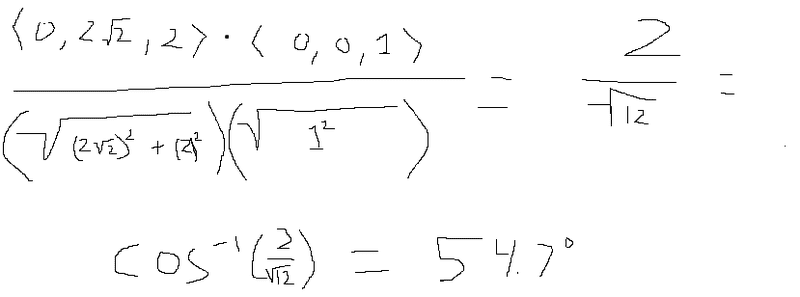# Equation of normal line and tangent plane

## Homework Statement

A cup is represented by the surface -(z-1)2 + x2 + y2 = 1
and it is on a table represented by the plane z=0

a) find the angle at which the cup intersects the table

b) find the equation of the normal line to the cup at the point (0, √2 , 2)

c) find the equation the tangent plane at the point (0, √2 , 2)

## The Attempt at a Solution

for a) I use cos θ = a⋅b / |a||b|

a and b are normals of the cup and table at the point of intersect, which are found by finding the gradients.

∇ƒcup = <Fx, Fy, Fz> = < 2x , 2y, -2(z-1) >

∇ƒtable = <Fx, Fy, Fz> = < 0 , 0 , 1 >

so at the point of contact between the cup and table, z is 0.

plugging that into the equation of the surface of the cup :

-(0-1)2 + x2 + y2 = 1

x2 + y2 = 2

so the intersection between the cup and table is a circle with radius √2

then I find a point on the circle x2 + y2 = 2 , setting x = 0 then y = √2
then a point is (0,√2, 0)

plugging this point into < 2x , 2y, -2(z-1) > and < 0 , 0 , 1 >:

< 0, 2√2, 2 > and < 0 , 0 , 1 >

∴ cos θ = a⋅b / |a||b|

b) plugging the point (0,√2, 2) into < 2x , 2y, -2(z-1) >:

< 0, 2√2, -2 >

equation of the normal line to the cup at point (0,√2, 2) =

(0,√2, 2) + t < 0, 2√2, -2 > = ( 0 , √2 + 2√2 t , 2-2t )

c) equation of tangent plane at point (0,√2, 2) :

2√2(y- √2) -2(z-2) = 0

can someone check my work?

#### Attachments

•upload_2015-11-5_19-10-54.png
14.4 KB · Views: 633
Last edited:

ehild
Homework Helper

## Homework Statement

A cup is represented by the surface -(z-1)2 + x2 + y2 = 1
and it is on a table represented by the plane z=0

a) find the angle at which the cup intersects the table

b) find the equation of the normal line to the cup at the point (0, √2 , 2)

c) find the equation the tangent plane at the point (0, √2 , 2)

## The Attempt at a Solution

for a) I use cos θ = a⋅b / |a||b|

a and b are normals of the cup and table at the point of intersect, which are found by finding the gradients.

∇ƒcup = <Fx, Fy, Fz> = < 2x , 2y, -2(z-1) >

∇ƒtable = <Fx, Fy, Fz> = < 0 , 0 , 1 >

so at the point of contact between the cup and table, z is 0.

plugging that into the equation of the surface of the cup :

-(0-1)2 + x2 + y2 = 1

x2 + y2 = 2

so the intersection between the cup and table is a circle with radius √2

then I find a point on the circle x2 + y2 = 2 , setting x = 0 then y = √2
then a point is (0,√2, 0)

plugging this point into < 2x , 2y, -2(z-1) > and < 0 , 0 , 1 >:

< 0, 2√2, 2 > and < 0 , 0 , 1 >

∴ cos θ = a⋅b / |a||b|

View attachment 91386

b) plugging the point (0,√2, 2) into < 2x , 2y, -2(z-1) >:

< 0, 2√2, -2 >

equation of the normal line to the cup at point (0,√2, 2) =

(0,√2, 2) + t < 0, 2√2, -2 > = ( 0 , √2 + 2√2 t , 2-2t )

c) equation of tangent plane at point (0,√2, 2) :

2√2(y- √2) -2(z-2) = 0

can someone check my work?
You forgot to take the square root when calculating the angle.

•catch22
You forgot to take the square root when calculating the angle.

#### Attachments

Last edited:
ehild
Homework Helper
It is not correct yet. √12 is not 4.

It is not correct yet. √12 is not 4.sorry, it is late over here :(

ehild
Homework Helper
It is right now!

•catch22
It is right now!
b and c are correct as well?

ehild
Homework Helper
b and c are correct as well?
They are correct but you can simplify the equation of the tangent plane.

They are correct but you can simplify the equation of the tangent plane.
√2(y- √2) -(z-2) = 0

ehild
Homework Helper
Expand the parentheses. What will be the resultant constant?

Expand the parentheses. What will be the resultant constant?
√2y- 2 - z + 2 = 0

√2y - z = 0

ehild
Homework Helper
It is much nicer, isn't it?